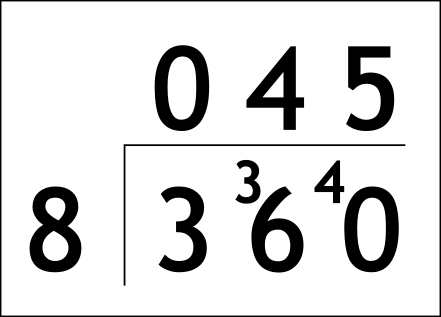The Singing Hedgehog Guide to:
Decimal Division 1

Here we have to remember one of the magic phrases:

FRACTIONS IS DIVISION,
DIVISION IS FRACTIONS

Here is a simple example.

36 ÷ 0.8

We don't know how to divide by 0.8, so we don't!

If we rewrite this as a fraction:

36
0.8

we can simply multiply top and bottom by ten to give:

360
8

Now we simply divide as normal by the
bus stop methodBecause we did the "same to the top as we did to the bottom"
of our fraction this IS the final answer.

next page

decimal index